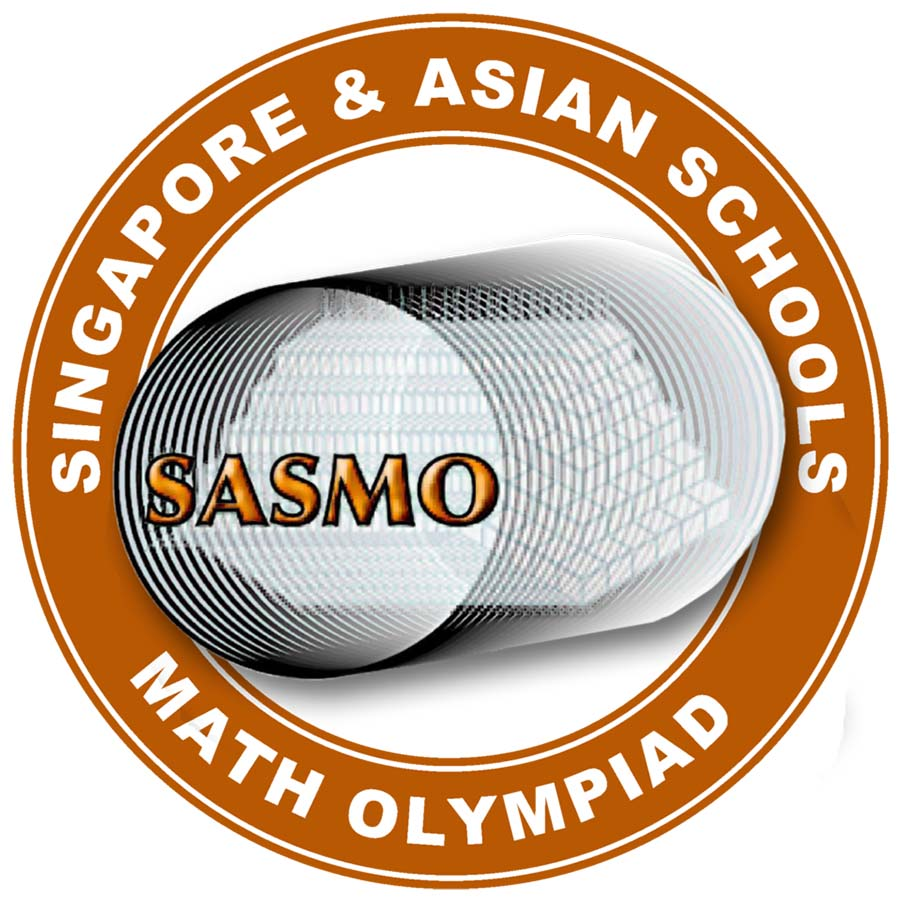## SASMO Test Preparation

### Unlimited Printable Worksheets, Online Practice and Online Tests For SASMOYou can use Edugain to prepare for SASMO exam using unlimited printable worksheets, online practice and online tests. For official information about SASMO, visit sasmo.sg

According to the website:

SASMO is a math olympiad contest that stretches not only the brightest students but is aimed at instilling confidence for all students.
The contest is designed in a way to make every student love Math again. SASMO contest fits nicely into the school curriculum with a high focus on non routine problem sums ‐ preparing for SASMO contest helps students prepare for the PSLE and O-Level exams.

*Edugain has no affiliation with SASMO or sasmo.sg. Edugain is a learning platform to help students prepare well for the exam.

### Grade 2 SASMO Preparation

Money Transactions, Large Numbers, Geometry, Multiplication, Division, Fractions, Measurement - Length, Mass & Capacity, Natural and Whole Numbers, Time, Data Handling, Numbers, Pictograph, Seasons, Calendar, Counting, Pattern, Mixed Operations, Logical Reasoning, Basic, Comparing Quantities

### Grade 3 SASMO Preparation

Mixed Operations, Logical Reasoning, Time, Shapes and Patterns, Large Numbers, Geometry, Multiplication, Roman Numerals, Division, Fractions, Measurement - Length, Mass & Capacity, Natural and Whole Numbers, Mensuration - Perimeter, Area, Volume, etc., Money Transactions, Symmetry, Graphs, Number System, Basic, Quadrilaterals, Numbers, Century Year, Tangram, Pictograph, Tally Marks, Data Handling

### Grade 4 SASMO Preparation

Large Numbers, Roman Numerals, Multiplication, Division, Mixed Operations, Unitary Method, Geometry, Natural and Whole Numbers, Fractions, Measurement - Length, Mass & Capacity, Decimals, Time, Mensuration - Perimeter, Area, Volume, etc., Logical Reasoning, Money Transactions, Symmetry, Graphs, Numbers, Tangram, Tiling, estimation

### Grade 5 SASMO Preparation

Large Numbers, Roman Numerals, Multiplication, Division, Mixed Operations, Unitary Method, Natural and Whole Numbers, Fractions, Decimals, Geometry, Measurement - Length, Mass & Capacity, Ratio & Proportions, Percentages, Time, Money Transactions, Mensuration - Perimeter, Area, Volume, etc., Logical Reasoning, Number System, Computation Operations, Data Handling, Graphs, Numbers, Pictograph, Simplification

### Grade 6 SASMO Preparation

Large Numbers, Natural and Whole Numbers, Integers, Fractions, Decimals, Ratio & Proportions, Percentages, Geometry, Mensuration - Perimeter, Area, Volume, etc., Algebra, Data Handling, Logical Reasoning, Unitary Method, Symmetry, Number System, Linear Equations In One Variable, Simplification, Triangles, Quadratic Equations, Numbers

### Grade 7 SASMO Preparation

Algebra, Integers, Symmetry, Data Handling, Logical Reasoning, Fractions, Mensuration - Perimeter, Area, Volume, etc., Geometry, Exponents and Powers, Decimals, Rational Numbers, Percentages, Ratio & Proportions, Congruence of Triangles, Heron's Formula

### Grade 8 SASMO Preparation

Algebra, Rational Numbers, Square and Square Roots, Graphs, Cubes and Cube Root, Exponents and Powers, Mensuration - Perimeter, Area, Volume, etc., Data Handling, Unitary Method, Percentages, Logical Reasoning, Natural and Whole Numbers, Linear Equations In One Variable, Geometry, Ratio & Proportions, Integers, Symmetry, Comparing Quantities, Polygons, Fractions

### Grade 9 SASMO Preparation

Coordinate Geometry, Algebra, Mensuration - Perimeter, Area, Volume, etc., Data Handling, Number System, Geometry, Heron's Formula, Quadrilaterals, Logical Reasoning, Linear Equations in Two Variables, Rational Numbers, Real Numbers, Congruence of Triangles, Euclid's Geometry , Constructions , Area, Percentages, Quadratic Equations

### Grade 10 SASMO Preparation

Trigonometry, Arithmetic Progressions, Data Handling, Real Numbers, Logical Reasoning, Quadratic Equations, Linear Equations in Two Variables, Algebra, Mensuration - Perimeter, Area, Volume, etc., Geometry, Time, Coordinate Geometry, CIRCLE, Euclid's Geometry , Triangles, Constructions , Probability, Complex Numbers, Logarithm, Geometric Progression, Circles and Parabola, Slope, Functions, Permutation And Combination

Articles on Olympiad exam preparation:

Back to top